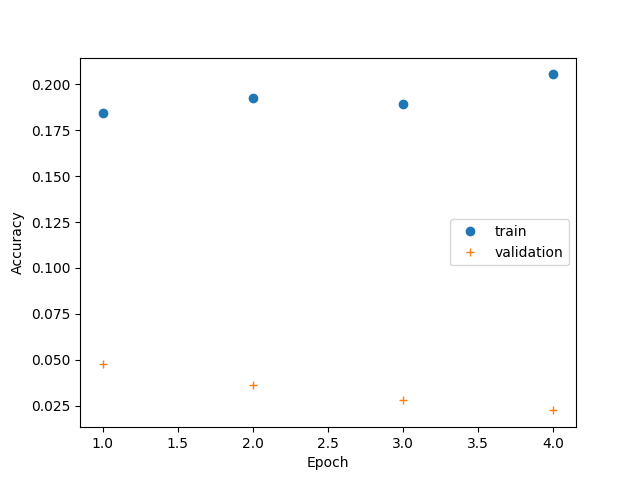# Plotting Neural Network Training Curves¶

This is a basic example using a convolutional recurrent neural network to learn segments directly from time series data

```# Author: David Burns

import matplotlib.pyplot as plt
import numpy as np
from tensorflow.python.keras.layers import Dense, LSTM, Conv1D
from tensorflow.python.keras.models import Sequential
from tensorflow.python.keras.wrappers.scikit_learn import KerasClassifier
from pandas import DataFrame
from sklearn.model_selection import train_test_split

from seglearn.pipe import Pype
from seglearn.transform import Segment
```

## Simple NN Model¶

```def crnn_model(width=100, n_vars=6, n_classes=7, conv_kernel_size=5,
conv_filters=3, lstm_units=3):
input_shape = (width, n_vars)
model = Sequential()

metrics=['accuracy'])

return model
```

## Setup¶

```# load the data
X = data['X']
y = data['y']

# split the data
X_train, X_test, y_train, y_test = train_test_split(X, y, test_size=0.25, random_state=42)

# create a segment learning pipeline
pipe = Pype([('seg', Segment(width=100, step=100, order='C')),
('crnn', KerasClassifier(build_fn=crnn_model, epochs=4, batch_size=256,
verbose=0, validation_split=0.2))])
```

Out:

```/home/circleci/miniconda/envs/testenv/lib/python3.8/site-packages/sklearn/utils/validation.py:67: FutureWarning: Pass memory=None as keyword args. From version 0.25 passing these as positional arguments will result in an error
warnings.warn("Pass {} as keyword args. From version 0.25 "
```

## Accessing training history¶

```# this is a bit of a hack, because history object is returned by the
# keras wrapper when fit is called
# this approach won't work with a more complex estimator pipeline, in which case
# a callable class with the desired properties should be made passed to build_fn

pipe.fit(X_train, y_train)
history = pipe.history.history
print(DataFrame(history))

# depends on version
if 'accuracy' in history:
ac_train = history['accuracy']
ac_val = history['val_accuracy']
elif 'acc' in history:
ac_train = history['acc']
ac_val = history['val_acc']
else:
raise ValueError("History object doesn't contain accuracy record")

epoch = np.arange(len(ac_train)) + 1
```

Out:

```/home/circleci/miniconda/envs/testenv/lib/python3.8/site-packages/seglearn/transform.py:237: VisibleDeprecationWarning: Creating an ndarray from ragged nested sequences (which is a list-or-tuple of lists-or-tuples-or ndarrays with different lengths or shapes) is deprecated. If you meant to do this, you must specify 'dtype=object' when creating the ndarray
Xt = np.array([sliding_tensor(Xt[i], self.width, self._step, self.order)
loss  ...  val_accuracy
0  2.004245  ...      0.098039
1  1.992355  ...      0.103641
2  1.981575  ...      0.100840
3  1.973452  ...      0.100840

[4 rows x 4 columns]
```

## Training Curves¶

```plt.plot(epoch, ac_train, 'o', label="train")
plt.plot(epoch, ac_val, '+', label="validation")
plt.xlabel("Epoch")
plt.ylabel("Accuracy")
plt.legend()
plt.show()
```Total running time of the script: ( 0 minutes 6.692 seconds)

Gallery generated by Sphinx-Gallery